# Notes from my studies: Recurrent Neural Networks and Long Short-Term Memory

This post contains notes from my studies. It’s mostly to keep them where I can find them, but if someone else finds them useful, that’s good too ☺️.

Before we kick off, here’s what I look like to trying to fix LaTeX when it’s pasted into Medium:Photo by Siora Photography on Unsplash

Back in June 2019 I wrote a 10 page report on the inner workings of recurrent neural networks and long-short term memory units. Not as fun as implementing them, but useful nonetheless!

Background

An artificial neural network (ANN) is a network of interconnected algorithms used in machine learning to recognise patterns in data. Neural networks learn in a supervised way — they ’learn’ patterns from training samples and are able to perform predictive tasks such as classification or regression.

The basic neural network architecture is the feedforward neural network. The data moves forward through the network, starting at an input layer, through any hidden layers, to an output layer. Here, we will focus on a variant to this basic structure called Recurrent Neural Networks (RNNs), which introduces a feedback loop to allow the output of the network to be fed back into the network as a new input.

The addition of a feedback loop allows the network to process, learn and predict sequential data. Traditional feedforward networks cannot do this because inputs and outputs to these networks are vectors of fixed size, assumed to be independent from each other. For example, if you train a network to recognise images of cats and dogs, and use it to classify ten images, the prediction for each image is not affected by the outcome for the previous, or any other, image. Sequential data is different; it can vary in length and the values are not independent, the data points are related to the data that has come before them.

With the feedback loop modification, RNNs can process every element in a sequence in turn and persist information added by each element. This gives RNNs the capability to handle temporal dependancies, and so can process sequences of vectors of variable length as both input and output, to perform such tasks as:

• Predicting the next word in a sentence.

Prior to the advent of RNNs, solving predictive tasks relating to sequential data was achieved with methods such as Hidden Markov Models (HMM). HMMs assign each element in a sequence a class, and compute probabilities that the next item in a sequence will be that class, then will select the most likely class as its prediction. Although HMMs are fast and efficient on shorter sequences, to achieve high accuracy on complex sequences (where a value is dependent on values many places behind it) they become exponentially expensive, and are outperformed by RNNs on these complex tasks .

The foundation for RNNs was led by research in the 1980’s, with ‘Hopfield networks’ — a type of network with a memory . RNNs saw significant improvement in the 1990’s with the invention of Long Short-Term Memory (LSTMs) RNNs , which solved a major issue with standard RNNs, the vanishing/exploding gradient problem, discussed in the following section. A further development to the LSTM architecture was presented by Cho, et al. in 1994, the Gated Recurrent Unit (GRU) , also discussed below.

Today, RNNs in various forms are present in many household applications. Google’s Voice Search uses RNNs , the Apple personal assistant Siri uses GRUs in its speech synthesis  and the language translation abilities of Google Translate were improved in 2016 using a deep LSTM network .

Standard Recurrent Neural Networks

RNNs replace the hidden layer in a regular neural network with a recurrent layer (or layers), which introduce the propagation of data back into the network at each time step. The network state and output at each time step is therefore dependant on the previous time step.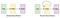Layers in a regular neural network (left) and a recurrent neural network (right).

Recurrent neural networks process a sequence of values u₁, . . . , with time step index t ranging from 1 to τ. Given an input vector ut and a neural network state xt, an output vector zt is produced at each time step t.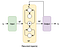Recurrent neural network architecture.

The current state of the neural network is dependant on the previous state and the current input, given by:

Where:

It follows that the output zt is not only dependant on the input, but the current state of the network, and therefore is also influenced by all previous inputs. A parametric function f ( . ) represents output layer computation, for a classification task this could be softmax, and for regression tasks this could be L2 norm squared error:

where Wo are the output layer weights.

The figure below shows an example of an RNN with one hidden layer that has been ‘unrolled’, illustrating the specific inputs and outputs at each time step.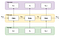Diagram of an ‘unrolled’ RNN.

Training an RNN

During training, the network is fed training samples, and produces a predicted output zt′ at each time step. In order to optimise the network, the back-propagation training algorithm is used.

During back-propagation, the error or ‘loss’ (the difference between predicted output zt′ with the target output from the training data zt) is measured using a loss function. The amount of contribution to this error that occurs at the final time step is calculated. Then the contribution from the layer before that and so on, until the input layer is reached. The propagation of the error backwards through the network allows the algorithm to measure the error gradient across all the network connections. Once the error gradients are known, gradient descent is used to tweak the weights and biases within the network so as to reduce the error. The training and updating of weights and biases continues to repeat, with the goal of learning the correct weights and biases to correctly predict the target output.

Back-propagation through time.

Unlike traditional ANNs which use separate weights for each hidden layer, RNNs share the weights across each time step — if the network is unrolled through time, it can be considered to have a separate layer at each time step, all sharing the same weight matrix. As the weights are shared across each time step, the gradient of the error at each step is dependant on the loss at all previous steps. For example, to calculate the gradient at step five, the gradients of the previous four steps are summed together with the gradient at step five. This is known as back propagation through time or BPTT.

Issues with RNNs: Vanishing and Exploding Gradient Problems

When summing the contributions of each time step to the gradient during BPTT, particularly for long sequences, the gradient values can suffer from the Vanishing Gradient Problem. This occurs if some of the weights are small; when summing, the subsequent gradient will keep on getting smaller and tend to ~0, or ‘vanish’. This is a problem because gradients are used during training to update the network parameters, so, as the gradient lowers, the parameter updates become insignificant and the network does not learn. This is not an issue for standard ANNs, as they don’t share parameters across the layers and no summing is necessary.

Similarly, if the weights are large then the final value would approach infinity, known as an Exploding Gradient. A simple and effective solution to the exploding gradient problem is to set a threshold to ‘clip’ the gradient value if it is too large. Countering the vanishing gradient problem is more difficult. One solution is to use the ReLU activation function in place of tanh or sigmoid activation functions, as the ReLU derivative is either 0 or 1 and so a vanishing gradient is less likely. Alternatively, a variation on standard RNNs called Long Short-Term Memory networks was developed specifically to address this problem.

Long Short-Term Memory Networks

Long Short-Term Memory (LSTM) networks, first proposed in 1997 by Hochreiter and Schmidhuber, advanced RNNs by addressing the Vanishing Gradient Problem  and are now commonly used. The structure of LSTMs is similar to a vanilla RNN, but replace the function that computes the hidden state with a cell. Cells accept as input the previous state xt−1 and the current data value ut and have the ability to keep or remove information to the state through the use of gates.

The standard RNN hidden layer is simple, such as a tanh layer.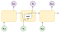Architecture of a standard RNN.

The recurrent layer in LSTM adds three sigmoid layers, termed ‘gates’. One to control what information is forgotten, the forget gate f, one to control which values to update, the input gate i, and a final layer that creates a new vector of candidate values that could be added to the state, the output gate o.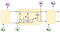Architecture of a LSTM network.

Each of these gates is a sigmoid neural net layer that outputs values between 0 and 1, controlling how much information should be passed through the gate. A value of 0 does not let any information pass, and a value of 1 lets all information pass when multiplying element-wise with another vector.

In order to update the cell state in a standard RNN, we use the following equation:

In order to update the cell state in LSTM, first the forget gate is used to decide what information to discard:

Where ft is the output of the forget gate, Wf is a weight matrix, zt−1 is the previous output, ut is the current input and bf is a bias vector. ft is comprised of a value between 0 and 1 for every value in the previous cell state xt−1, to control what to discard from the cell state. Next, there is a two part operation to decide what new information to add into the cell state. Firstly, the input gate decides which values in the cell state to update:

Where it is the output of the input gate, Wi is a weight matrix and bi is a bias vector. Secondly, a tanh layer creates new values that could be added to the cell state:

Where x ̃ is the output of the tanh layer, W is a weight matrix and b is a bias vector. Now it is possible to update the cell state using a combination of the outputs from the input gate, forget gate and tanh layer:

The previous state is multiplied by the output from the forget gate, to discard information. The new values to be added are scaled using the output from the input gate and added to the previous state, resulting in the new cell state xt. Now the cell state has been updated, the output from the network can be calculated. This is controlled by the output gate and another tanh layer. The output gate is given by:

Giving the final output from the network zt as:

The vanishing gradient problem is no longer an issue when back-propagating errors through time in LSTM networks. During back propagation, if the derivative of the output ∂xt is starting to converge to 0, the values of the forget gate can increase, preventing ∂xt−1 the gradients from from vanishing. As the values of f, o, i and x ̃ are learnt by the network, the network can decide when to let the gradient vanish and when not to do so. It does not matter how long the sequence is, the network has a mechanism for information not to be forgotten, and longer term dependences can be modelled.

Gated Recurrent Units

A popular variant on the LSTM network called the Gated Recurrent Unit (GRU) was introduced by Cho, et al. in 2014 . GRU cells are simplified versions of the LSTM cell, but have comparable performance.

A GRU has two gates, a reset gate and an update gate, which are very similar to the gates in LSTM. The update gate is a combination of LSTM’s input and forget gates, and decides how much of the previous cell state to persist. The reset gate, that decides how to combine the new data, is applied directly to the previous state.

Applications of Recurrent Neural Networks

It has been shown that RNNs and variants are well suited to processing sequential data, and one such application is the translation of video data into a semantic description of its contents.

The classification of images and videos is a fundamental challenge in the field of computer vision. Convolutional neural networks (CNNs) have achieved state of the art performance on image classification and when used in combination with RNNs, the task of providing video descriptions using neural networks has seen significant progress.

A neural network based approach to this task was described by Venugopalan, et al. . They posit that by using a CNN to label individual video frames, the output from the CNN can be used as input to an LSTM to generate a sentence describing the activity in the video, the architecture they developed is shown below: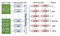The video description network architecture. Features are extracted for each frame, mean pooled across the video, and inputted to the LSTM network at each time step. The LSTM outputs one word at each time step, based on the video features (and the previous word) until it picks an end-of-sentence tag.

Several CNN-LSTM architectures were trained and evaluated on a dataset of over 1970 videos depicting an activity, with human-generated descriptions. Experiments found that a model pre-trained on large image datasets (of which there are more available than labelled video datasets) and then using transfer learning to train on video datasets performed better compared to model trained only on video descriptions.

Further work on automated video descriptions is being undertaken. An end-to- end network for activity recognition and video description was proposed by Donahue, et al. in 2016  also used a CNN-LSTM combination, and RNN-based solutions remain a popular approach to this problem .

References

1. Kostadinov, S., Recurrent Neural Networks with Python Quick Start Guide. 2018: Packt Publishing.

Senior Data Scientist @ UK Hydrographic Office

## More from Cate Seale

Senior Data Scientist @ UK Hydrographic Office